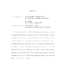## Long time stability of rotational Euler dynamics##### Files
umi-umd-4511.pdf(350.74 KB)
We study the stabilizing effect of rotational forcing in the nonlinear setting of two-dimensional shallow-water and Euler equations. We prove that when rotational force dominates the pressure, it prolongs the life-span of smooth solutions for $t\lesssim 1+\ln(\delta^{-1})$ where $\delta\ll 1$ is the ratio of the (inverse of) squared Froude number measuring the amplitude of pressure, relative to the (inverse of) Rossby number, measuring the dominant rotational force. The strong rotation also imposes certain periodicity to the flow in the sense that there exists a nearby'' periodic-in-time approximation of the exact solution. In the opposite regime of large $\delta$'s, the flow is dispersive so that the divergence field substantially decays in finite time and therefore periodicity is not retained.